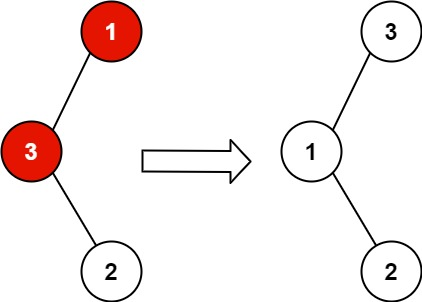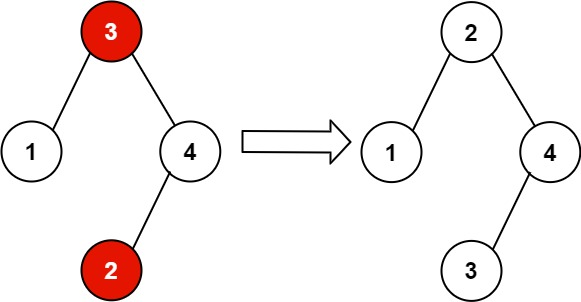# 99. Recover Binary Search Tree

You are given the `root` of a binary search tree (BST), where the values of exactly two nodes of the tree were swapped by mistake. Recover the tree without changing its structure.

Example 1:```Input: root = [1,3,null,null,2]
Output: [3,1,null,null,2]
Explanation: 3 cannot be a left child of 1 because 3 > 1. Swapping 1 and 3 makes the BST valid.
```

Example 2:```Input: root = [3,1,4,null,null,2]
Output: [2,1,4,null,null,3]
Explanation: 2 cannot be in the right subtree of 3 because 2 < 3. Swapping 2 and 3 makes the BST valid.
```

Constraints:

• The number of nodes in the tree is in the range `[2, 1000]`.
• `-231 <= Node.val <= 231 - 1`

## The solution to the above question is-

/**
* Definition for a binary tree node.
* struct TreeNode {
*     int val;
*     TreeNode *left;
*     TreeNode *right;
*     TreeNode() : val(0), left(nullptr), right(nullptr) {}
*     TreeNode(int x) : val(x), left(nullptr), right(nullptr) {}
*     TreeNode(int x, TreeNode *left, TreeNode *right) : val(x), left(left), right(right) {}
* };
*/
class Solution {
public:
TreeNode* f=NULL;
TreeNode* s=NULL;
TreeNode* prev=new TreeNode (INT_MIN);

void inorder(TreeNode* root){
if(root==NULL)return;

inorder(root->left);

if(f==NULL && prev->val>root->val)f=prev;

if(f!=NULL && prev->val > root->val)s=root;

prev=root;

inorder(root->right);
}

void recoverTree(TreeNode* root) {
inorder(root);
swap(f->val,s->val);
}
};